JEE  >  Physics Test 5 - Magnetic Effect, Magnetism, Electromagnetic Induction And Alternating Current

# Physics Test 5 - Magnetic Effect, Magnetism, Electromagnetic Induction And Alternating Current

Test Description

## 25 Questions MCQ Test JEE Main & Advanced Mock Test Series | Physics Test 5 - Magnetic Effect, Magnetism, Electromagnetic Induction And Alternating Current

Physics Test 5 - Magnetic Effect, Magnetism, Electromagnetic Induction And Alternating Current for JEE 2023 is part of JEE Main & Advanced Mock Test Series preparation. The Physics Test 5 - Magnetic Effect, Magnetism, Electromagnetic Induction And Alternating Current questions and answers have been prepared according to the JEE exam syllabus.The Physics Test 5 - Magnetic Effect, Magnetism, Electromagnetic Induction And Alternating Current MCQs are made for JEE 2023 Exam. Find important definitions, questions, notes, meanings, examples, exercises, MCQs and online tests for Physics Test 5 - Magnetic Effect, Magnetism, Electromagnetic Induction And Alternating Current below.
Solutions of Physics Test 5 - Magnetic Effect, Magnetism, Electromagnetic Induction And Alternating Current questions in English are available as part of our JEE Main & Advanced Mock Test Series for JEE & Physics Test 5 - Magnetic Effect, Magnetism, Electromagnetic Induction And Alternating Current solutions in Hindi for JEE Main & Advanced Mock Test Series course. Download more important topics, notes, lectures and mock test series for JEE Exam by signing up for free. Attempt Physics Test 5 - Magnetic Effect, Magnetism, Electromagnetic Induction And Alternating Current | 25 questions in 60 minutes | Mock test for JEE preparation | Free important questions MCQ to study JEE Main & Advanced Mock Test Series for JEE Exam | Download free PDF with solutions
 1 Crore+ students have signed up on EduRev. Have you?
Physics Test 5 - Magnetic Effect, Magnetism, Electromagnetic Induction And Alternating Current - Question 1

### An electron moving with kinetic energy 6.6 * 10-14J   enters a magnetic field 4 * 10-3T  at right angle to it.The radius of its circular path will be nearest to

Physics Test 5 - Magnetic Effect, Magnetism, Electromagnetic Induction And Alternating Current - Question 2

### A closely wound flat circular coil of 25 turns of wire has diameter of 10 cm which carries current of 4 A, the flux density at the centre of a coil will be

Detailed Solution for Physics Test 5 - Magnetic Effect, Magnetism, Electromagnetic Induction And Alternating Current - Question 2

Flux density = 2 * π * N * i/10r
or,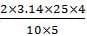= 12.56G or 1.25 * 10-3T

Physics Test 5 - Magnetic Effect, Magnetism, Electromagnetic Induction And Alternating Current - Question 3

### An electron is travelling along the x-direction. It encounters a magnetic field in the y-direction. Its subsequent motion will be

Detailed Solution for Physics Test 5 - Magnetic Effect, Magnetism, Electromagnetic Induction And Alternating Current - Question 3 Because a force of q(v×B) is acted on e⁻ toward +z axis that will change the direction of e⁻ and then it will move in a circle in x-z plane
Physics Test 5 - Magnetic Effect, Magnetism, Electromagnetic Induction And Alternating Current - Question 4
The magnetic field due to a straight conductor of uniform cross-section of radius a and carrying a steady current is represented by
Physics Test 5 - Magnetic Effect, Magnetism, Electromagnetic Induction And Alternating Current - Question 5

A cyclotron is operating at a frequency of  12 * 106 Hz. Mass of deuteron is 3.3 * 10-27 kg  and charge on deuteron is 1.6 * 10-19  induction of the neccessary magnetic field is

Physics Test 5 - Magnetic Effect, Magnetism, Electromagnetic Induction And Alternating Current - Question 6

The strength of the transverse magnetic field required to bend photoelectrons of maximum kinetic energy with in a circle of radius 50 cm when light of wavelength 4000Å is incident on barium emitter is (work function of barium is 2.5 eV)

Detailed Solution for Physics Test 5 - Magnetic Effect, Magnetism, Electromagnetic Induction And Alternating Current - Question 6

key idea: Body performing circular motion is always acted upon by a centripetal force provided by magnetic field. If the kinetic energy of photoelectrons emitted from the metal surface is E1 and W the work function them from Einstein's photoelectric equation, we have Eh = hv -W where v is frequency and h the Planck's constant
∴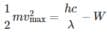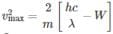Putting the numercial values from the question, we have
∴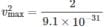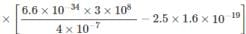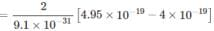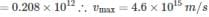A body performing circular motion is acted upon by a force directed towards its centre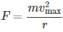Also the magnetic force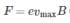On equating.
We have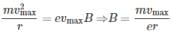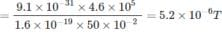Physics Test 5 - Magnetic Effect, Magnetism, Electromagnetic Induction And Alternating Current - Question 7
The semicircular portion (radius R) of a wire carrying current i is placed in a uniform magnetic field B. The magnetic field is perpendicular to the plane of the circle. The magnetic of the force on the wire is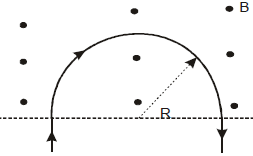Physics Test 5 - Magnetic Effect, Magnetism, Electromagnetic Induction And Alternating Current - Question 8
A & B are two concentric circular conductors of centre O and carrying currents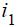and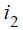as shown in the diagram. If the ratio of their radii is 1:2 and ratio of the flux densities at O due to A and B is 1:3, then the value of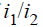will be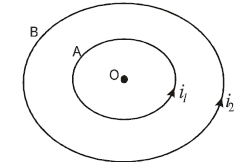Physics Test 5 - Magnetic Effect, Magnetism, Electromagnetic Induction And Alternating Current - Question 9
Domain formation is the necessary feature of
Physics Test 5 - Magnetic Effect, Magnetism, Electromagnetic Induction And Alternating Current - Question 10
The direction of the null points is on the equation line of a bar magnet, when the north pole of the magnet is pointing
Physics Test 5 - Magnetic Effect, Magnetism, Electromagnetic Induction And Alternating Current - Question 11
Two magnets each of magnetic moment M are placed so as to form a cross at right angles to each other. The magnetic moment of the system will be
Physics Test 5 - Magnetic Effect, Magnetism, Electromagnetic Induction And Alternating Current - Question 12

At a certain place, the horizontal component of earth’s magnetic field is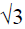times of its vertical component. the angle of dip at that place is

Physics Test 5 - Magnetic Effect, Magnetism, Electromagnetic Induction And Alternating Current - Question 13

A magnet makes 30 oscillations per minute in the earth’s magnetic field. If the magnetic field is doubled. The period of oscillation of magnet is

Detailed Solution for Physics Test 5 - Magnetic Effect, Magnetism, Electromagnetic Induction And Alternating Current - Question 13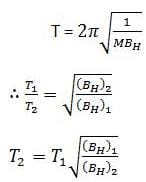n1 = 30 oscillation/min
n1 = 1/2  oscillation/s
T1 = 2s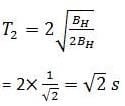Physics Test 5 - Magnetic Effect, Magnetism, Electromagnetic Induction And Alternating Current - Question 14
A moving conductor coil produces an induced emf. This is in accordance with
Physics Test 5 - Magnetic Effect, Magnetism, Electromagnetic Induction And Alternating Current - Question 15
The varying current in a coil changes from 10 A to zero in 0.5 s. If the average emf induced in the coil is 220 V, then the self-inductance of the coil will be
Detailed Solution for Physics Test 5 - Magnetic Effect, Magnetism, Electromagnetic Induction And Alternating Current - Question 15 Avg E= L(∆i/∆t) 220=L (10/0.5) 220=L(20) L=11H
Physics Test 5 - Magnetic Effect, Magnetism, Electromagnetic Induction And Alternating Current - Question 16
Two circular coils can be arranged in any of the three situations shown in the figure. Their mutual inductance will be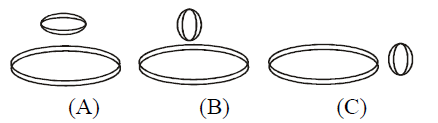Physics Test 5 - Magnetic Effect, Magnetism, Electromagnetic Induction And Alternating Current - Question 17

In the circuit shown in the figure, what is the value of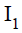just after pressing the key K?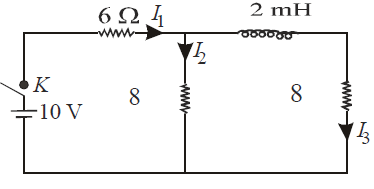Physics Test 5 - Magnetic Effect, Magnetism, Electromagnetic Induction And Alternating Current - Question 18
A uniform but time-varying magnetic field B(t) exists in cylindrical region of radius a and is directed into the plane of the paper, as shown. The magnitude of the induced electric field at point P at a distance r from the centre of the circular region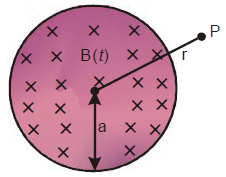Detailed Solution for Physics Test 5 - Magnetic Effect, Magnetism, Electromagnetic Induction And Alternating Current - Question 18
Construct a concentric circle of radius r. The induced electric field (E) at any point on the circle is equal to that at P. For this circle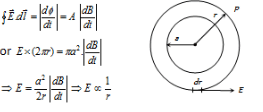Physics Test 5 - Magnetic Effect, Magnetism, Electromagnetic Induction And Alternating Current - Question 19
A device for generating and alternating current of a desired frequency is known as
Physics Test 5 - Magnetic Effect, Magnetism, Electromagnetic Induction And Alternating Current - Question 20
In an AC circuit containing only capacitance, the current
*Answer can only contain numeric values
Physics Test 5 - Magnetic Effect, Magnetism, Electromagnetic Induction And Alternating Current - Question 21

A long solenoid has 100 turns/meter and a current of 3.5 A is flowing through it. If it is filled with material of relative permeability (μr = 20) then magnetic field within the solenoid will be xπ × 10–4, find x?

Detailed Solution for Physics Test 5 - Magnetic Effect, Magnetism, Electromagnetic Induction And Alternating Current - Question 21

B = µ0µRni

*Answer can only contain numeric values
Physics Test 5 - Magnetic Effect, Magnetism, Electromagnetic Induction And Alternating Current - Question 22

A bar magnet is freely suspended in such a way that, when it oscillates in the horizontal plane. It makes 20 oscillations per minute at a place, where dip angle is 30° and 15 oscillations per minute at a place, where dip angle is 60°. Ratio of total earth's magnetic field at these two places is x : 9√3, find x :-

Detailed Solution for Physics Test 5 - Magnetic Effect, Magnetism, Electromagnetic Induction And Alternating Current - Question 22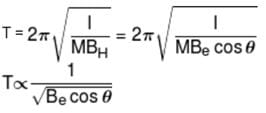*Answer can only contain numeric values
Physics Test 5 - Magnetic Effect, Magnetism, Electromagnetic Induction And Alternating Current - Question 23

A square loop ABCD carrying a current i, is placed near and coplanar with a long straight conductor XY carrying a current i, the net force on the loop will be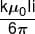Find the value of k.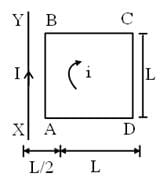Detailed Solution for Physics Test 5 - Magnetic Effect, Magnetism, Electromagnetic Induction And Alternating Current - Question 23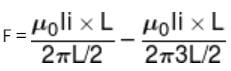*Answer can only contain numeric values
Physics Test 5 - Magnetic Effect, Magnetism, Electromagnetic Induction And Alternating Current - Question 24

A square metal wire loop of side 10 cm and resistance 1Ω moved with a constant velocity v in a uniform magnetic field B = 2T as shown in figure. The magnetic field is perpendicular to the plane of the loop and directed into the paper. The loop is connected to a network of resistors, each equal to 3Ω. What should be the speed of the loop in m/s so as to have a steady current of 1mA in the loop?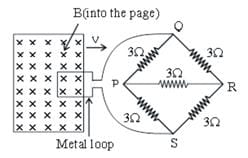Detailed Solution for Physics Test 5 - Magnetic Effect, Magnetism, Electromagnetic Induction And Alternating Current - Question 24

The given network PQRS is balanced Wheatstone's bridge. Hence the resistance connected in the diagonal arm PR becomes ineffective. The net effective resistance of the network is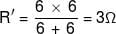The total resistance of the loop + network is
R = 1 + 3 = 4Ω
Induced current in the network,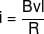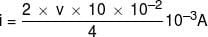v = 2 × 10–2 m/s = 2 cm/s

*Answer can only contain numeric values
Physics Test 5 - Magnetic Effect, Magnetism, Electromagnetic Induction And Alternating Current - Question 25

An AC source of angular frequency ω is fed across a resistor R and a capacitor C in series. The current registered is I. If now the frequency of source is changed to ω/3 (but maintaining the same voltage), the current in the circuit is found to be halved. The ratio of reactance to resistance at the original frequency ω is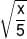then find the value of x.

Detailed Solution for Physics Test 5 - Magnetic Effect, Magnetism, Electromagnetic Induction And Alternating Current - Question 25

At angular frequency ω, the current in RC circuit is given by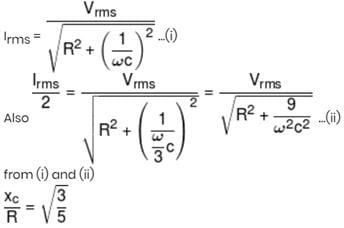## JEE Main & Advanced Mock Test Series

2 videos|327 docs|203 tests
 Use Code STAYHOME200 and get INR 200 additional OFF Use Coupon Code
Information about Physics Test 5 - Magnetic Effect, Magnetism, Electromagnetic Induction And Alternating Current Page
In this test you can find the Exam questions for Physics Test 5 - Magnetic Effect, Magnetism, Electromagnetic Induction And Alternating Current solved & explained in the simplest way possible. Besides giving Questions and answers for Physics Test 5 - Magnetic Effect, Magnetism, Electromagnetic Induction And Alternating Current, EduRev gives you an ample number of Online tests for practice

## JEE Main & Advanced Mock Test Series

2 videos|327 docs|203 tests